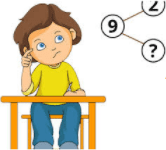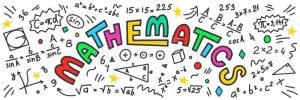# Year 2

Our Year 2 teachers are Mrs Charles (2CC) and Mrs Nolan (2AN)

Our support assistants are Mrs Porter and Miss Rasab

Autumn 1 2021There are some links on the Home Learning page.

Thank you for your continued support.

Mrs Charles and Mrs Nolan

Handwriting. You must be able to form your letters, sitting on the line correctly. Remember finger spaces between words.

HandwritingArithmetic

Can you complete these 15 questions?

 1. 5 + 5 + 5= 6. 2 x 7 = 11. 6 x 10 = 2. 4 + 3 + 7 = 7. 50 + 3 = 12. 17 + 2 = 3.52 – 30= 8. 67 – 7 = 13. 7 x 10 = 4. 39- ? = 10 9. ? – 5 = 8 14. 50 ÷ 10 = 5. 8 x 10 = 10. 23 + 21 = 15. 10 ÷ 10=Learn By HeartLearn by Heart HomeworkThe Autumn term focus:

Place Value

Make your own resources to support your learning. The children are familiar using counters, place value counters, tens and ones, arrow cards and 100 squares in school. Download and print the document below.

Addition and subtraction activities week 1